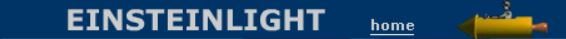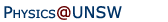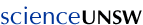Relativity in brief... or in detail..

## The weirdness - and the logic - of the principle of invariance of the speed of light

• How weird is the invariance of the speed of light?,
• Light, electromagnetic waves and the logic of relativity.
• Galileo's principle of relativity and Einstein's principle of Special Relativity.

How weird is the invariance of the speed of light? Is the principle of Special Relativity really counter-intuitive?

It depends on how one expresses it, and to whose intuition one appeals. For example, it does seem counter-intuitive that the speed of light does not depend on the motion of the observer. In our animation, Zoe turns on the headlights of her space ship. She measures the speed of light from her headlights as c with respect to her. Jasper sees her travelling towards him at (let's say) v. He measures the speed of light from her headlights as c. No, not c+v, but just c. Surely this is counter-intuitive? Maybe even crazy? Surely relative speeds add up? Let's consider first two more familiar cases.Again, Zoe travels towards Jasper at speed v. First, suppose that, instead of light, Zoe fires an arrow from her car at speed u, as she measures it. Jasper will measure its speed as v+u, as predicted by Galilean relativity. Alternatively, if she sounds the horn, the sound will travel forward with a speed vsound with respect to the air that is the medium for the sound wave. In the absence of wind, Jasper will measure the sound to be travelling at vsound, and Zoe will measure its speed relative to her as vsound−v, again as specified by Galilean relativity. It is tempting to extrapolate one or other of these results to light: if light is like little arrows, Jasper should measure v+c. If light is like sound, and if its medium is stationary with respect to Jasper, than Zoe should measure its speed as c−v. (We discuss the possibility of a medium for light later.) If we are really careful in our thinking, however, we should also say that light may be neither like arrows nor like sound.

The extrapolation mentioned above is tempting, but it would be a huge extrapolation: light travels nearly a million times faster than sound. And extrapolations are always dangerous. For instance, very near where you are now, the temperature and other physical conditions are (I hope) fairly comfortable. But the further you go from your familiar surroundings — suppose for instance you go 20 kilometers up or down — the more likely things are to be different in surprising ways. The further we go from the familiar, the more likely we shall be surprised. And the speed of light is a very unfamiliar speed.

Indeed, the speed of light (about 300,000 km/s — over a billion k.p.h.) is so great that our intuition is of little use. All the observations about speed that you have ever made, all of the experience upon which your common sense is based, are in a tiny area of physical reality that we could label "extremely low speed" compared with light. It is often the case that one can make approximations that apply over a limited region of reality, but that fail when we examine a larger range. For instance, objects fall at 9.8 metres per second per second in the lab. Also in the basement or on the roof. But this is not true in high orbits, or at the centre of the Earth.

At this stage, many of my students say things like "The invariance of the speed of light among observers is impossible" or "I can't understand it". Well, it's not impossible. It's even more than possible, it is true. This is something that has been extensively measured, and many refinements to the Michelson and Morely experiment, and complementary experiments have confirmed this invariance to very great precision. As to understanding it, there isn't really much to understand. However surprising and weird it may be, it is the case. It's true in our universe. The fact of the invariance of c may require some accepting but it doesn't take much understanding: what requires understanding are its consequences, and how it can be integrated into what we already know.

But let's pursue the logic. Can we explain why the universe is this way? In physics, explanations go deeper and deeper, but they have to stop somewhere: laws of nature are our basic building blocks. For instance, if you ask why the electric force follows an inverse square law, we can give a subtle explanation that goes one level further down: in a sense, Gauss' law shows that the inverse square law is a result of the geometry of a three dimensional universe. (Some students will then say "very well, but I might as well just accept that it is an inverse square law and stop there".) Similarly, we shall now go further with the principle of Special Relativity, because we can give some insight into why the Universe is this way. (But again, you might find it more comfortable just to accept the invariance as a law of nature.)

For physicists, the principle of Special Relativity (SR) seems elegant and almost necessary, even though most of us still feel that it is counter-intuitive in some ways. Partly this is due to the familiarity that physicists have with SR, but mainly it is due to our familiarity with electromagnetism. Let's now see how the principle of SR is not necessarily counter-intuitive, but follows logically from the (nicely intuitive) principle of Galilean relativity.

### Light, electromagnetic waves and the logic of relativity

A wave phenomenon arises directly from the equations for electricity and magnetism (Maxwell's equations). On the way, the speed of light appears in terms of two constants, one electric and one magnetic. This is presented somewhat more formally on Maxwell's equations: are they really so beautiful that you would dump Newton?, but here is a qualitative or "arm-waving" explanation:

Vibrations and waves require two things. For example, a mass on the end of a spring oscillates because, when disturbed, the spring pulls it back towards its resting postion. However, when the mass gets to its resting position, it is now moving rapidly because of the action of the spring. So, because of its inertia, it overshoots. From that moment, the spring now slows it down. When it stops, the spring is stretched, so it accelerates the mass back towards its equilibrium position. Once there, it overshoots. Voilà: oscillation.

Let's generalise this idea. First, there must be an effect that acts to return the system towards equilibrium. For a wave in a stretched guitar string, it is the tension in the string that tends to straighten it out. For sound, it is the elastic property of the medium: squeeze it, and it 'bounces back', expand it and it contracts. (The relevant quantity for air — the 'springiness' of air for rapid changes — is about 1.4 times its pressure.)

Next there must something with inertia: something that, once moving, tends to keep on moving (like the moving mass in our mass and spring example). For a wave in a stretched string, the inertia arises because each small length of string has a certain mass. Here the relevant quantity is the mass per unit length of the string. For a sound wave in air, each small volume has mass and the inertia comes from the density of the air. (By the way, here are links for more about waves on strings and on sound waves in pipes.)

What is it about space — about pure vacuum — that allows it to carry electromagnetic waves? Taking a few liberties, we could say that the electric field is in a sense elastic or springy, in that it acts on the things that cause it (charge) to pull them back to equilibrium (Coulomb's or Gauss' law), while a magnetic field is inherently a bit like inertia because it acts to maintain the things that cause it, which are currents (Faraday's and Lenz' laws).

For all of these waves, we can work out the speed without actually producing a wave. For a string, we could measure the tension and mass per unit length of a string; for sound we would need the pressure (strictly, the adiabatic modulus) and density of the gas. In fact, for all waves like this, the speed turns out to be just the square root of the ratio of the "springiness" to the "inertial property".

Similarly we can work out the speed of light by putting charges on metal conductors and measuring the electric field produced, and by passing a current through a coil or wire and measuring the magnetic field it produces. (A common experiment in physics teaching laboratories measures the speed of light almost exactly this way, as does our electric circuit example.)

### Galileo's principle of relativity and Einstein's principle of special relativity

Now let's compare the principles of relativity of Galileo and Einstein.

Galilean relativity implies that, if a physicist on a station platform performs a mechanical experiment, and another in a smoothly passing train performs the same experiment, the two obtain identical results. (The laws of mechanics are the same in two different inertial frames.)

Einstein's principly of relativity is just like Galileo's, except that now the experiment can be either a mechanical experiment or an electromagnetic experiment. The physicist on the train measures the electric field produced by putting charges on metal conductors and the magnetic field produced by a current in a coil of wire. She will get the same result with her apparatus as would another physicist, on the platform, with similar equipment. Einstein's principle of relativity is just a generalisation of that of Galileo and Newton.

If you forget about the source of the light, a light wave passing is just an experiment involving electric and magnetic fields and so its speed must be that given by the electricity and magnetism experiments. Experimentally, we find that the speed of the source does affect some things about the wave (its wavelength changes: the Doppler effect) but it doesn't influence the speed.

In our animations in module 1, the juggler who uses the same laws of mechanics on the platform and on a smoothly moving train demonstrates Galilean relativity. But when you board a train, you might also expect that any electromagnetic devices that you bring should also work in the moving frame.

So, to someone who is familiar with electromagnetism, the principle of special relativity is, at least in this sense, quite intuitive: it is reasonable that the physicist on the train should get the same electric field from the same charge, and the same magnetic field from the same current, as does the colleague on the platform.

From the similarities of electrical and magnetic experiments conducted by physicists in different frames it follows that an electromagnetic wave will have the same speed when measured by either physicist, regardless of its source. From this (and a few other reasonable propositions) the results of special relativity follow.

This is an informal account. It is possible to derive the speed of light from the one dimensional or integral versions of Maxwell's equations using only high school maths. Such versions are presented in introductory physics texts, and the more interested lay reader should be able to follow these with a little effort. We give a treatment on Maxwell's equations: are they really so beautiful that you would dump Newton?.

For more about the experiments, see also But is it true? Is the speed of light really independent of the motion of the observer?

### Back to the invariance of the speed of light. What about that animation?

Well you are probably thinking "even if I accept that, how can I explain the animation? How can Jasper and Zoe's 'speedometers' both get the same value of the speed of light? Just looking at it, I can see that the red dot is approaching Jasper faster than it is leaving Zoe. How do they get the same answer?

The answer is time dilation. (Go to the film clip or to the detailed page about time dilation.) We are looking at this animation from Jasper's frame of reference and, according to Jasper, time runs slowly for Zoe. Zoe's clocks, including the timing mechanism of her 'speedometer', run slowly. So Zoe records the same value for the speed of light.

The previous paragraph inverts the usual order of presentation of these ideas. The more fundamental and precise experiments show that the speed of light is independent of the observer, and from this we deduce time dilation. However, we also observe time dilation directly, and so we could use this to deduce that the speed of light is independent of the observer.

For a discussion of the "variation of the speed of light with time or place", see Beyond Relativity 2: Is the speed of light really constant?

•Home | Summary | Quiz | Credits School of Physics - The University of New South Wales Sydney, Australia 2052 © School of Physics UNSW 2052 Disclaimer feedback